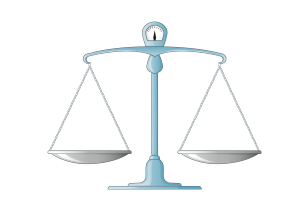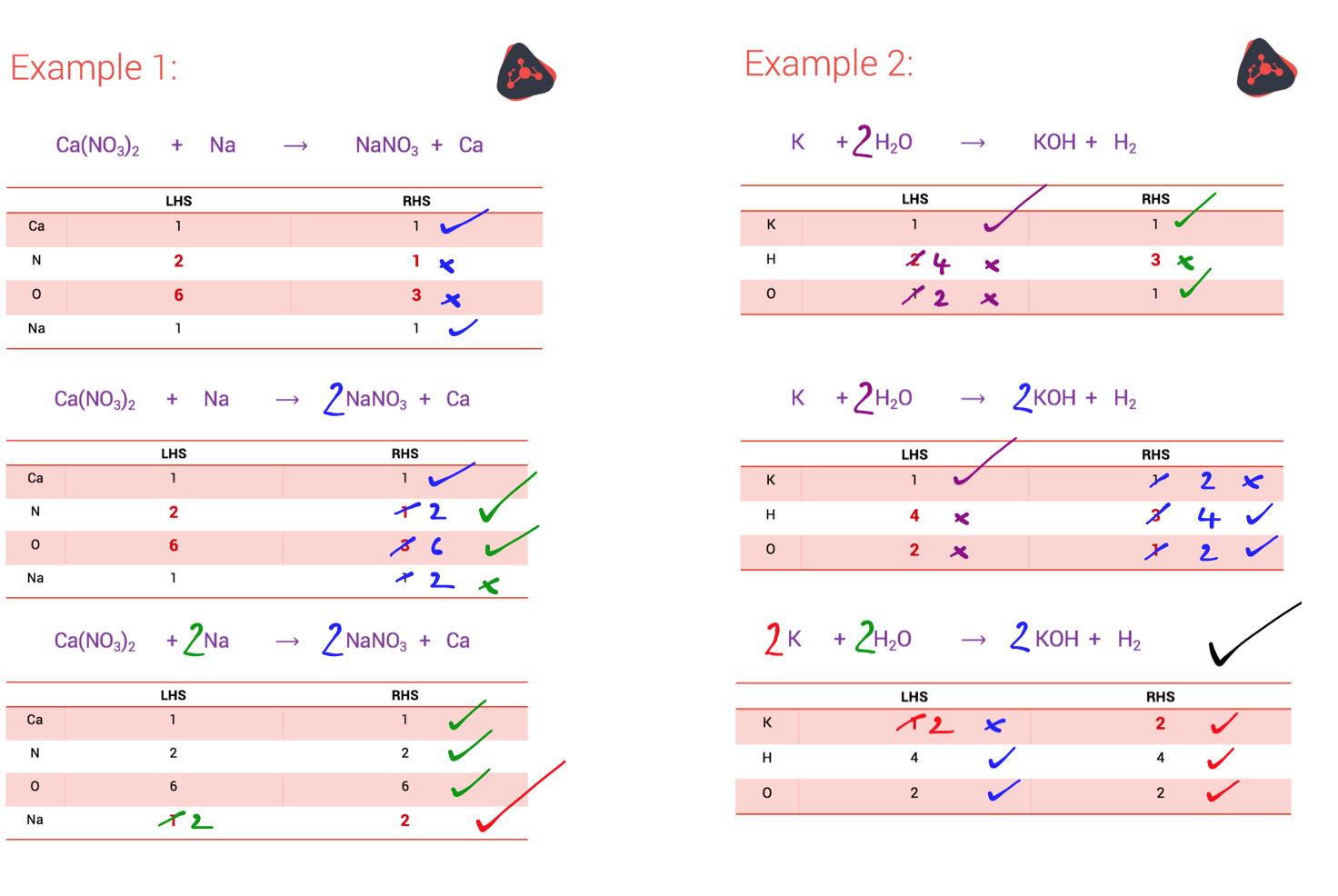Chemistry 24/10/2018

# Balancing chemical equations

By LIGAYA BATTEN

Balancing chemical equations can look a bit daunting and it scares a lot of students. But don’t be afraid… Below we show you a straightforward method to balance equations that you can rely on every time.

## The law of conservation of mass and balancing chemical equationsFirst, let’s briefly discuss the theory.

The law of conservation of mass says that in a chemical reaction:

must equal

### the mass of the reactants

So what does this mean, exactly? In practical terms it means that:

the number of atoms of each element on the left-hand side (LHS) of a chemical equation

must equal

the number of atoms of each element on the right-hand side (RHS) of a chemical equation

Often, this is easy enough to check. For example, take the simple equation below:

C + O2    ⟶    CO2

This is clearly a balanced equation: there’s one carbon and two oxygen atoms on the LHS and the same on the RHS. So the equation is balanced.

But sometimes, the answer isn’t obvious. There are several ways to approach a difficult equation. Our favourite method is to use a table to tally the number of atoms of each element on the LHS of the equation and the RHS of the equation.

## Using a table to balance equations

The steps are:

• If the number of each atom on either side is the same, you’re all good, and the equation is balanced.
• If the number of each atom on either side is NOT the same, you need to use big numbers in front of each element or compound to even them out.
• Every time you add a big number, you multiply the whole compound by that number, and so you adjust the numbers in the table accordingly.
• Repeat until you have the same number of every atom on the LHS as on the RHS.

The best way to learn how this system works is to practise the steps. We’ve given two examples below of how it works – check them out.NOTE: You can only use big numbers to balance equations, not the small numbers you use when you’re working out the chemical formula for the compound.

For more discussion on balanced equations, have a look at our videos on this topic: AQA , Edexcel and OCR. Each video takes you through detailed worked examples.

No surprise: for this topic especially, lots of practice really helps. Check out the worked examples in the video. Then try our exam-style question on balancing equations, and check your answer against our answer scheme.

Happy balancing!

Ligaya Batten